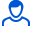Content: 6.1.2.jpg (20.58 KB)

Positive responses: 0
Negative responses: 0

Sold: 11
Refunds: 0Loyalty discount! If the total amount of your purchases from the seller more than:

 \$1 the discount is 1% \$5 the discount is 2% \$10 the discount is 3% \$20 the discount is 5% \$50 the discount is 7% \$100 the discount is 10% \$200 the discount is 15%

6.1.2
Bracket ABD consists of homogeneous rods AB and BD with the same linear weight. What should be the length in cm of the rod AB so that the xc center of gravity of the bracket equals zero if BD = 20 cm. (Answer 28.3)
No feedback yet